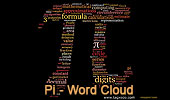# Word Cloud of Pi, Archimedes' constant and related topicsWord Cloud of Pi and related topics
π (Pi) is a mathematical constant whose value is the ratio of any circle's circumference to its diameter in Euclidean space; this is the same value as the ratio of a circle's area to the square of its radius. It is approximately equal to 3.141593 in the usual decimal notation. Many formulae from mathematics, science, and engineering involve π, which is one of the most important mathematical and physical constants. Unlike many physical constants, pi is a dimensionless quantity, meaning that it is simply a number without physical units. Source: Wikipedia, Pi.

Word Cloud
Word cloud or tag cloud is a graphical representation of word frequency. Presents a picture of the most common words used with those used more often displayed larger and are typically listed alphabetically.
Word cloud generator software: Tagxedo.comHome | Search | Software | Word Cloud | Word Cloud: Math | Geometry Word Cloud | Geometry | Pi | Pi Day 2015: Celebrating 12 Digits of Pi | Post a comment | Email